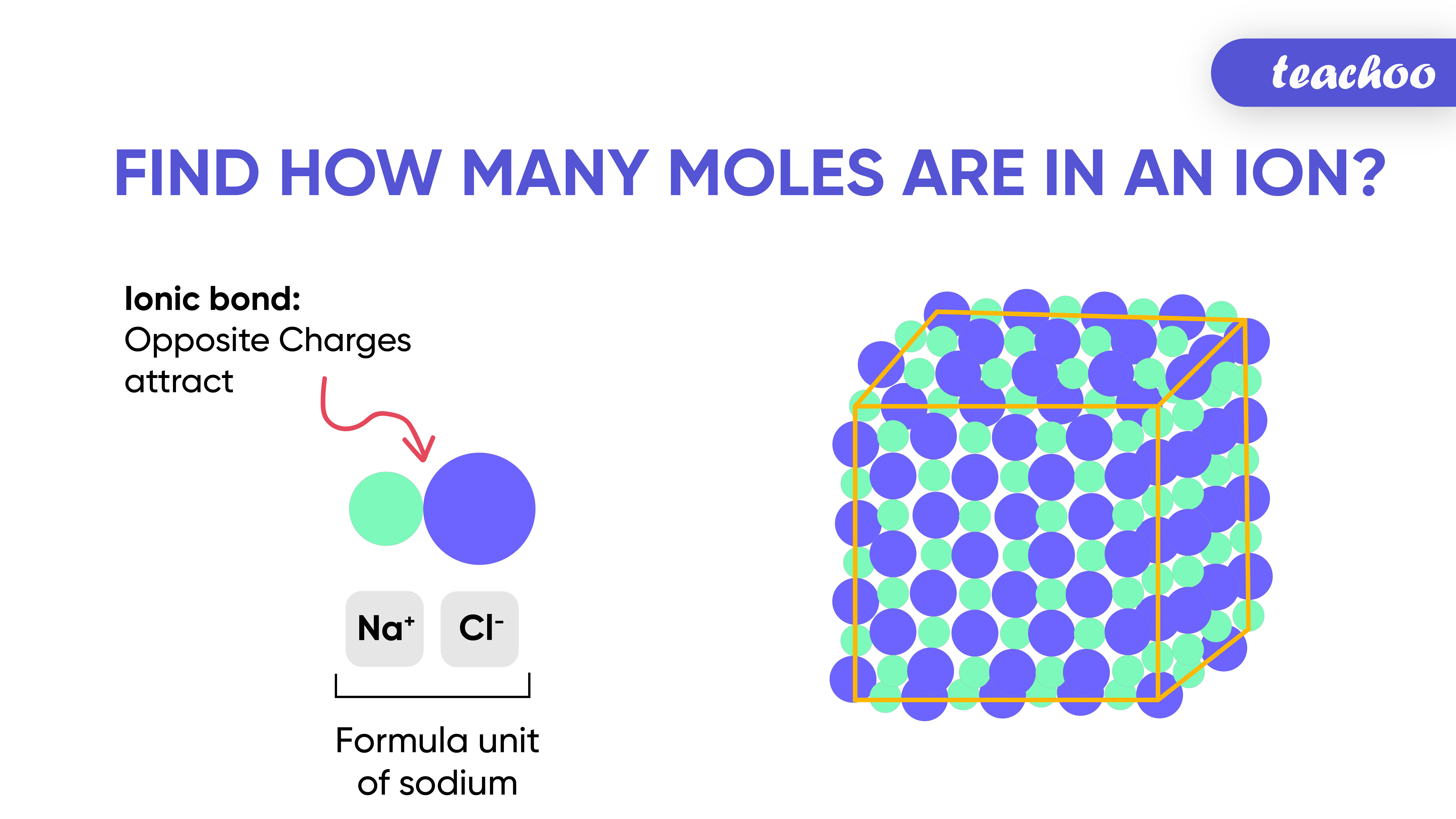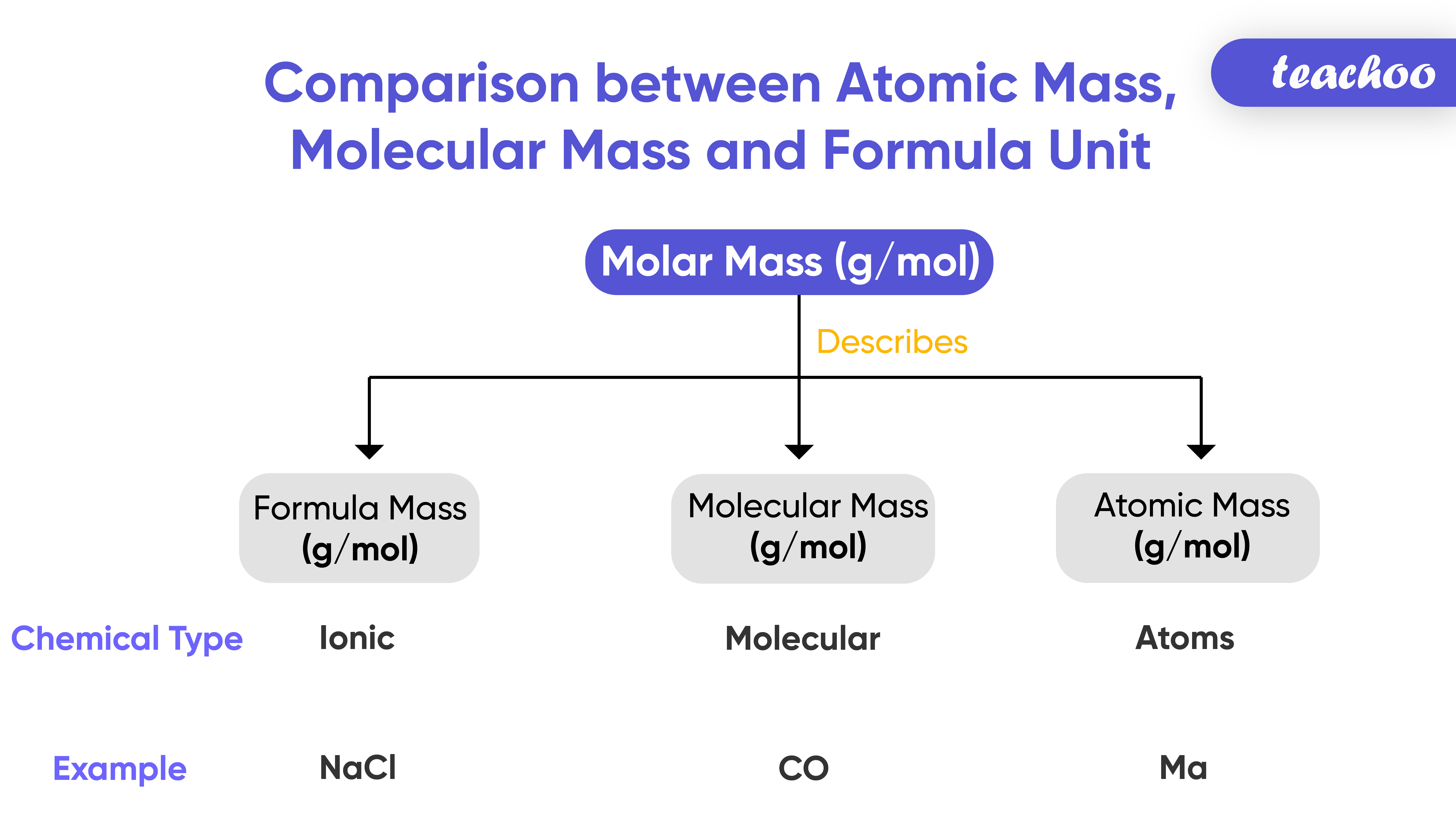# What is Molecular Mass?

It is the sum of atomic mass of all the atoms in a molecule

Example - Molecular Mass of H 2 O

• Atomic Mass of Hydrogen (H) =1 u
• Atomic Mass of Oxygen (O) =16 u
• Molecular Mass of Water (H 2 O) = 2*1+16*1 = 2 + 16 = 18 u

Example - Molecular Mass of HNO 3

• Atomic Mass of Hydrogen (H) =1 u
• Atomic Mass of Nitrogen (N) =14 u
• Atomic Mass of Oxygen (O) =16 u
• Molecular Mass of Nitric Acid (HNO 3 ) = 1 *1+ 14*1+ 16*3 = 1+14 + 48 = 63 u

A few more examples of Molecular masses are:

## What is a Molecule?

A group of atoms chemically bonded together is Here are the valency of a few elements:

called Molecule.

## What is Formula Unit Mass?

It is sum of atomic mass of all atoms in formula unit of compound

It is calculated in same manner as Molecular Mass

Only difference is it is used for those substances whose constituent element is ions

(it is used for ionic compounds)## What is a Molecule?

A group of atoms chemically bonded together is Here are the valency of a few elements:

called Molecule.

## Example 1

Sodium Chloride (NaCl) contains 2 ions, Positively charged Sodium ions (Na+) and Negatively charged Chloride Ions

Formula Unit Mass of Sodium Chloride

Atomic Mass of Sodium (Na) = 23 u

Atomic Mass of Chloride (Cl)= 35.5 u

Formula Unit Mass of Sodium Chloride = 23*1+ 35.5*1 = 23+35.5 = 58.5 u

## What is a Molecule?

A group of atoms chemically bonded together is Here are the valency of a few elements:

called Molecule.

## Example 2

Calcium Chloride ( CaCl 2 ) contains 2 IONS, Positively charged Calcium ions (Ca +2 ) and Negatively charged Chloride (2 Cl -1 ) Ions

Formula Unit Mass of Calcium Chloride

Atomic Mass of Calcium (Ca) = 40 u

Atomic Mass of Chloride (Cl)= 35.5 u

Formula Unit Mass of Calcium Chloride  (CaCl 2 ) = 40*1 + 35.5*2 = 40+71 = 111 u

## What is a Molecule?

A group of atoms chemically bonded together is Here are the valency of a few elements:

called Molecule.

## Comparison between Atomic Mass, Molecular Mass and Formula Unit MassAtomic Mass Molecular Mass Formula Unit Mass It is the mass of an atom of a chemical element It is the Sum of Atomic Masses of all the atoms in a molecule It is sum of atomic mass of all atoms in formula unit of compound It is used to measure mass of a single atom It is used to measure mass of a single molecule It is used to measure mass of those substances whose constituent elements are ions

## What is a Molecule?

A group of atoms chemically bonded together is Here are the valency of a few elements:

called Molecule.

 Atomic Mass of Hydrogen = 1 u Molecular Mass of Water (H 2 O) = (2x1) +(16x1)  =2+1= 18 u Formula Unit Mass of Sodium Chloride (NaCl) = (23x1) + (35.5x1) =23+35.5 = 58.5 u

Example - 3.1,3.2

In text Question - Page 40 Q1,Q2

NCERT Question -

1. Class 9
2. Chapter 3 Class 9 - Atoms And Molecules (Term 2)
3. Concepts

Concepts

Class 9
Chapter 3 Class 9 - Atoms And Molecules (Term 2)